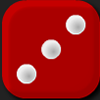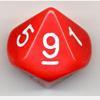Search by Topic

Resources tagged with Rounding similar to Round the Four Dice:

Filter by: Content type:
Age range:
Challenge level:

There are 6 results

Broad Topics > Numbers and the Number System > RoundingRound the Four Dice

Age 7 to 11 Challenge Level:

This activity involves rounding four-digit numbers to the nearest thousand.Round the Dice Decimals 2

Age 7 to 11 Challenge Level:

What happens when you round these numbers to the nearest whole number?Round the Three Dice

Age 7 to 11 Challenge Level:

What happens when you round these three-digit numbers to the nearest 100?Round the Dice Decimals 1

Age 7 to 11 Challenge Level:

Use two dice to generate two numbers with one decimal place. What happens when you round these numbers to the nearest whole number?Rough Rectangle

Age 11 to 14 Short Challenge Level:

What is the smallest possible area that this rectangle could have?Reasoned Rounding

Age 7 to 14 Challenge Level:

Four strategy dice games to consolidate pupils' understanding of rounding.